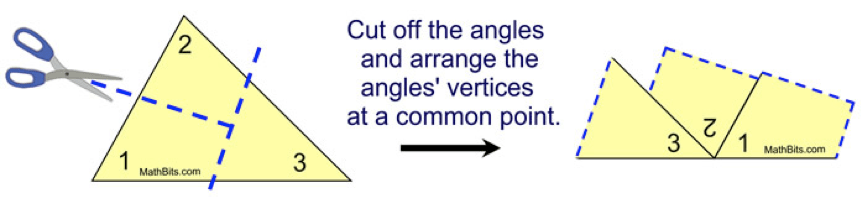# GEOM 2 | Lesson 1 | Watch (Triangle Sum Theorem)# Triangle Sum Theorem

Obtain a printed copy of a large scalene triangle, or draw one on a sheet of paper. Cut the triangle out, and tear off each of the three corners. Move the corners so that they form a line, as such:“What is the angle measure of a line, or straight angle? If all 3 angles from the triangle formed the line, then what can you say about the sum of the angles?”

Watch this video if necessary and remember to return to this page by using the back arrow.

Triangle Sum Theorem Proof